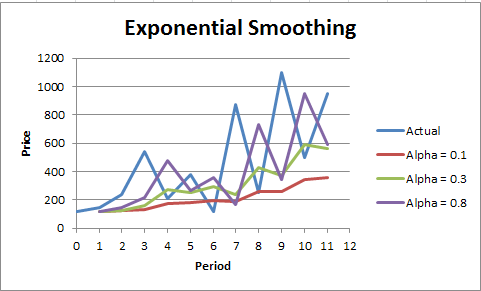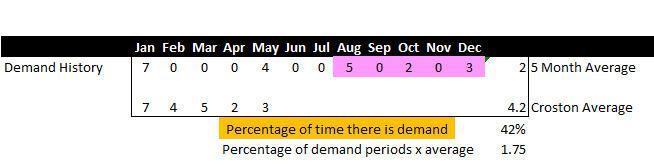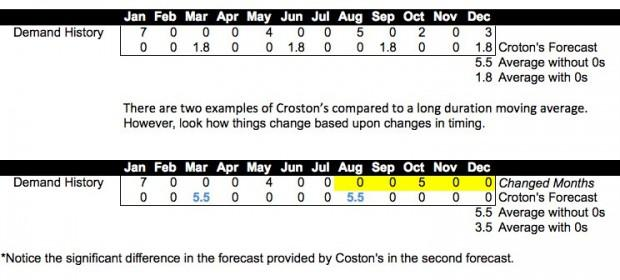# 5 Statistical Methods For Forecasting Quantitative Time Series

Time is one of most important factors on which our businesses and real life depends. But, technology has helped us manage the time with continuous innovations taking place in all aspects of our lives. Don’t worry, we are not talking about anything which doesn’t exist. Let’s be realistic here!

Here, we are talking about the techniques of predicting & forecasting future strategies. The method we generally use, which deals with time-based data that is nothing but “Time Series Data” & the models we build ip for that is “Time Series Modeling”. As the name indicates, it’s basically working on time (years, days, hours, and minutes) based data, to explore hidden insights of the data and trying to understand the unpredictable nature of the market which we have been attempting to quantify.

TIME SERIES:

The time series data used to provide visual information to the unpredictable nature of the market we have been attempting to quantify and trying to get a grip on that.

An Ordered sequence of observations of a variable or captured object at equally distributed time interval. Time series is anything which is observed sequentially over the time at regular interval like hourly, daily, weekly, monthly, quarterly etc. Time series data is important when you are predicting something which is changing over the time using past data. In time series analysis the goal is to estimate the future value using the behaviours in the past data.

There are many statistical techniques available for time series forecast however we have found few effectives ones which are listed below:

Techniques of Forecasting:

• Simple Moving Average (SMA)
• Exponential Smoothing (SES)
• Autoregressive Integration Moving Average (ARIMA)
• Neural Network (NN)
• Croston

METHOD-I: SIMPLE MOVING AVERAGE (SMA)

Introduction:

A simple moving average (SMA) is the simplest type of technique of forecasting. Basically, a simple moving average is calculated by adding up the last ‘n’ period’s values and then dividing that number by ‘n’. So the moving average value is considering as the forecast for next period.

Why Do We Use SMA?

Moving averages can be used to quickly identify whether selling is moving in an uptrend or a downtrend depending on the pattern captured by the moving average.

i.e. A moving average is used to smooth out irregularities (peaks and valleys) to easily recognize trends.

SMA Working Example:

Let us suppose, we have a time series data, to have a better understanding on SMA, Where, we have the graphical view of our data, in that we have twelve observations of Price with equal interval of time. After plotting our data, it seems that it has upward trend with lot of peaks and valleys.Conclusion: The larger the interval, the more the peaks, and valleys are smoothed out. The smaller the interval, the closer the moving averages are to the actual data points. The SMA is basically deal with historical data having more and more peak and valleys. Probably it would be stock data, retail data etc.

METHOD-II: EXPONENTIAL SMOOTHING

Introduction:

This is the second well known method to produce a smoothed Time Series. Exponential Smoothing assigns exponentially decreasing weights as the observation get older.

Why Do We Use Exponential Smoothing?

Exponential smoothing is usually a way of “smoothing” out the data by removing much of the “noise” (random effect) from the data by giving a better forecast.

Types of Exponential Smoothing Methods

• Simple Exponential Smoothing: –

If you have a time series that can be described using an additive model with constant level and no seasonality, you can use simple exponential smoothing to make short-term

forecast.

• Holt’s Exponential Smoothing: –

If you have a time series that can be described using an additive model with increasing or decreasing trend and no seasonality, you can use Holt’s exponential smoothing to make

short-term forecasts.

• Winters’ Three Parameter Linear and Seasonal Exponential Smoothing: –

If you have a time series that can be described using an additive model with increasing or decreasing trend and seasonality, you can use Holt-Winters exponential smoothing to make short-term forecasts.

Graphical Views:

Exponential Smoothing:Here, we have alpha value that is smoothing constant and this method is called simple exponential smoothing method which consider other two factors as constant (i.e. Seasonality & Trend). Double’s (Holt’s) Exp. Smoothing & Winter’s Exp. Smoothing Methods dealing two factors i.e. Trend and Seasonality (i.e. Beta & Gamma).

Conclusion: Larger the alpha, closer to the actual data points and vice versa. This method is suitable for forecasting data with no trend or seasonal pattern (alpha = Smoothing Constant).

METHOD-III AUTOREGRESSIVE INTEGRATED MOVING AVERAGE (ARIMA)

Autoregressive Integrated Moving Average (ARIMA):

A statistical technique that uses time series data to predict future. The parameters used in the ARIMA is (P, d, q) which refers to the autoregressive, integrated and moving average parts of the data set, respectively. ARIMA modeling will take care of trends, seasonality, cycles, errors and non-stationary aspects of a data set when making forecasts.

Understanding ARIMA Model in General Term: –

How to Understand ARIMA model?

To understand this, we can refer real time scenario that is sugar cane juicer, from juicer it is difficult to extract all the juice in one go, so the shopkeeper repeats the process for several times till there is no more juice left in the residual. That’s how ARIMA works, the idea with ARIMA models is that the final residual should look like white noise otherwise there is juice or information available in the data to extract.

How Do We Use ARIMA Model?

ARIMA checks stationarity availability in the data, the data should also show a constant variance in its fluctuations over time. To get the proper information about the parameter used in ARIMA is based on “identification process” which was purposed by Box-Jenkins.

When Do We Use ARIMA Model?

As we all know ARIMA is mainly used to project future values using historical time series data. Its main application is in short forecasting with minimum 38-40 historical data points with minimum number of outliers. If you do not have at least 38 data points, then it advisable to look for some other methods.

Working Example of ARIMA

Here, we are trying to understand ARIMA using quarterly European retail trade data from 1996 to 2011. The data are clearly non-stationary, with some seasonality, so we will first take a seasonal difference. The seasonally differenced data are shown in Fig. These also appear to be non-stationary, and so we take an additional first difference and may be next if require. Shown in Fig.As we considered seasonal ARIMA model which first checks their basic requirements and is ready for forecasting. Forecasts from the model for the next three years are shown in Figure. Notice how the forecasts follow the recent trend in the data (this occurs because of the double differencing).

Conclusion: – It works best when your data exhibits a stable or consistent pattern over time with a minimum amount of outliers.

METHOD-IV NEURAL NETWORK

Introduction:

ANN: – Artificial neural network (ANN) is basically machine learning approach that models human brain and consists of a number of artificial neurons. Their ability to learn by example makes them very flexible and powerful.

Why Do We Use Neural Networks?

Neural networks, has its own strength to derive meaning from complicated or imprecise data, and most of the time can be used to detect the pattern and trend in the data, which cannot be detectable easily from human eye or any computer techniques. We also have some of the advantage of NN like Adaptive learning, self-organization, real-time operation, fault tolerance.

Applications of neural networks

Now a day, in every field NN is equally important, for example, some of the fields I have listed below: –

• Sales Forecasting

• Industrial Process Control

• Customer Research

• Data Validation

• Risk Management

• Target Marketing

Conclusion:

We can use NN in any type of industry and get benefited, as it is very flexible and also doesn’t requires any algorithms. They are regularly used to model parts of living organisms and to investigate the internal mechanisms of the brain.

METHOD-V CROSTON

Introduction:

It’s modification of exponential smoothing for sporadic demand product time series suggested by Croston in 1972. The core value of this method is not only the estimation of average demand volume, but also estimation of time interval length between two non-zero demands, term called as intermittent demand.

The Croston method works in two steps, First, separate exponential smoothing estimates are made of the average size of a demand. Second, the intermittent demands are calculated. This is then used in a form of the constant model to predict the future demand.

How Croston’s Work?

Croston’s has a complex formula, however, what it output is actually very simple. The screenshot below explains what Croston’s does in a very simple way for the sake of understanding.Above is the 12-month average vs. Croston’s vs, while below is the 5-month average vs. Croston’s.As you can see, Croston’s removes the periods that have no demand only averaging the periods that have demand. Next Croston’s calculates the frequency of the demand. The math behind this is complex, but the output is extremely similar to performing an exponential smoothing.

Why Do We Use CROSTON’s?

In the given fig. we have two Croston’s forecast based on demand histories, with more non-zero data-points. Here Croston’s will come into the picture and show its benefits.• At the very beginning, Croston’s starts detecting cyclic and periodicity into the data points of demand patterns. In this case, it is suggested that a demand could occur possibly after 3.5 (4 after roundup) zero period.

• The second most important thing which Croston’s does is, it adjusts the next occurrence from the last non-zero period, if the recent periods are zero periods.

So the objects of forecast are predicting the consumption at the right moment with right quantity. Croston’s does try to predict the “right moment”, which is more sophisticated than the moving average.

Conclusion:

The Croston method is a forecast strategy for products with intermittent demand. In the univariate forecast profile, choose forecast strategy.

Croston’s can be easily emulated with exponential smoothing and any timing benefit is usually adjusted by order lot sizing, and or safety stock in supply planning. Therefore, demand history must not only be lumpy, but must also be very low in order for Croston’s to be of value. Therefore, Croston’s can be seen as a specialty forecasting method that provides value in certain limited circumstance.

For more information on the Statistical method for forecasting or any such type of implementation, you can simply reach out to us at feedback@bistasolutions.com. If you’d like to implement software with forecasting tools for your business, get in touch using our contact form.

•
•
•
•
•
•

2 Comments on "5 Statistical Methods For Forecasting Quantitative Time Series"

Notify ofGuest
Md Zishan Hussain
1 year 11 months ago
Hello there, I am given a time series data and I am using Neural Network for predicting the output variable. the Problem is as I am increasing the number of observation in training accuracy is decreased. As a matter of fact, the number of observation increases then accuracy increases. I… Read more »Guest
rameena abp
1 year 4 months ago
Drawing trend lines is one of the few easy techniques that really WORK. Prices respect a trend line, or break through it resulting in a massive move. Drawing good trend lines is the MOST REWARDING skill. The problem is, as you may have already experienced, too many false breakouts. You… Read more »